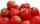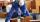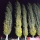# Together

Grandfather, father, and son are carpenters. They decided to make a wardrobe.
Father produces it for 12 hours, his son for 15 hours, and grandfather for 10 hours.
How long will they make it together?

Result

x =  4 h

#### Solution:

x*(1/12+1/15+1/10)=1

15x = 60

x = 4

Calculated by our simple equation calculator.

Leave us a comment of example and its solution (i.e. if it is still somewhat unclear...):

Showing 0 comments:Be the first to comment!#### To solve this example are needed these knowledge from mathematics:

Need help calculate sum, simplify or multiply fractions? Try our fraction calculator. Do you have a linear equation or system of equations and looking for its solution? Or do you have quadratic equation?

## Next similar examples:

1. StrawberriesFather collects strawberries himself in 4 hours, son in 14 hours. How long will it take them along when dad comes to help son collect strawberries after 3 hours?
2. EquationSolve the equation: 1/2-2/8 = 1/10; Write the result as a decimal number.
3. Unknown numberI think the number - its sixth is 3 smaller than its third.
4. Equation with fractionsSolve equation: ? It is equation with fractions.
5. Unknown numberIdentify unknown number which 1/5 is 40 greater than one tenth of that number.
6. Bricklayers8 bricklayers build a house for 630 days. How many bricklayers have to add after 150 days, so then the whole building completed in (next) 320 days?
7. Hectoliters of waterThe pool has a total of 126 hectoliters of water. The first pump draws 2.1 liters of water per second. A second pump pumps 3.5 liters of water per second. How long will it take both pumps to drain four-fifths of the water at the same time?
8. FractionsThree-quarters of an unknown number are 4/5. What is 5/6 of this unknown number?
9. UN 1If we add to an unknown number his quarter, we get 210. Identify unknown number.
10. Simple equationSolve for x: 3(x + 2) = x - 18
11. EqnSolve equation with fractions: 2x/3-50=40+x/4
12. Simple equation 6Solve equation with one variable: X/2+X/3+X/4=X+4
13. Cleaning windowsCleaning company has to wash all the windows of the school. The first day washes one-sixth of the windows of the school, the next day three more windows than the first day and the remaining 18 windows washes on the third day. Calculate how many windows ha
14. PearsThere were pears in the basket, I took two-fifths of them, and left six in the basket. How many pears did I take?
15. Equation with xSolve the following equation: 2x- (8x + 1) - (x + 2) / 5 = 9
16. Two workersThe first worker would himself completed the work in 8 hours, the other in 6 hours. After two hours of joint work left the first worker to a doctor and other completed work himself. How many hours worked the other worker himself?
17. PoplarHow tall is a poplar by the river, if we know that 1/5 of its total height is a trunk, 1/10th of the height is the root and 35m from the trunk to the top of the poplar?